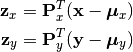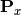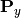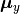Canonical Correlation Analysis¶

Canonical Correlation Analysis (CCA) is a statistical analysis technique to identify correlations between two sets of variables. Given two vector variables X and Y, it finds two projections, one for each, to transform them to a common space with maximum correlations.

The package defines a CCA type to represent a CCA model, and provides a set of methods to access the properties.

Properties¶

Let M be an instance of CCA, dx be the dimension of X, dy the dimension of Y, and p the output dimension (i.e the dimensio of the common space).

xindim(M)

Get the dimension of X, the first set of variables.

yindim(M)

Get the dimension of Y, the second set of variables.

outdim(M)

Get the output dimension, i.e that of the common space.

xmean(M)

Get the mean vector of X (of length dx).

ymean(M)

Get the mean vector of Y (of length dy).

xprojection(M)

Get the projection matrix for X (of size (dx, p)).

yprojection(M)

Get the projection matrix for Y (of size (dy, p)).

correlations(M)

The correlations of the projected componnents (a vector of length p).

Transformation¶

Given a CCA model, one can transform observations into both spaces into a common space, asHere,andare projection matrices for X and Y;andare mean vectors.

This package provides methods to do so:

xtransform(M, x)

Transform observations in the X-space to the common space.

Here, x can be either a vector of length dx or a matrix where each column is an observation.

ytransform(M, y)

Transform observations in the Y-space to the common space.

Here, y can be either a vector of length dy or a matrix where each column is an observation.

Data Analysis¶

One can use the fit method to perform CCA over given datasets.

fit(CCA, X, Y; ...)

Perform CCA over the data given in matrices X and Y. Each column of X and Y is an observation.

X and Y should have the same number of columns (denoted by n below).

This method returns an instance of CCA.

Keyword arguments:

name description default
method

The choice of methods:

• :cov: based on covariance matrices
• :svd: based on SVD of the input data
:svd
outdim The output dimension, i.e dimension of the common space min(dx, dy, n)
mean

The mean vector, which can be either of:

• 0: the input data has already been centralized
• nothing: this function will compute the mean
• a pre-computed mean vector
nothing

Notes: This function calls ccacov or ccasvd internally, depending on the choice of method.

Core Algorithms¶

Two algorithms are implemented in this package: ccacov and ccasvd.

ccacov(Cxx, Cyy, Cxy, xmean, ymean, p)

Compute CCA based on analysis of the given covariance matrices, using generalized eigenvalue decomposition.

Parameters: Cxx – The covariance matrix of X. Cyy – The covariance matrix of Y. Cxy – The covariance matrix between X and Y. xmean – The mean vector of the original samples of X, which can be a vector of length dx, or an empty vector Float64[] indicating a zero mean. ymean – The mean vector of the original samples of Y, which can be a vector of length dy, or an empty vector Float64[] indicating a zero mean. p – The output dimension, i.e the dimension of the common space. The resultant CCA model.
ccasvd(Zx, Zy, xmean, ymean, p)

Compute CCA based on singular value decomposition of centralized sample matrices Zx and Zy.

Parameters: Zx – The centralized sample matrix for X. Zy – The centralized sample matrix for Y. xmean – The mean vector of the original samples of X, which can be a vector of length dx, or an empty vector Float64[] indicating a zero mean. ymean – The mean vector of the original samples of Y, which can be a vector of length dy, or an empty vector Float64[] indicating a zero mean. p – The output dimension, i.e the dimension of the common space. The resultant CCA model.